CivilBay

Home  > Steel  > Base Plate Design - CISC SectionToolTip :

BASE PLATE DESIGN

Result Summary
Overall         ratio = 0.75 OK

Design Code Reference
Base plate design based on                 Code Abbreviation
AISC Design Guide 1: Base Plate and Anchor Rod Design 2nd Edition AISC Design Guide 1
CSA A23.3-14  Design of Concrete Structures CSA A23.3-14
Code Reference
Base Plate Data
Column section type   =
Column section size   =
Column section properties d = 308.0 [mm] tf = 15.4 [mm]
bf = 305.0 [mm] tw = 9.9 [mm]
Base plate anchor bolt pattern   =
Base plate anchor bolt pattern   =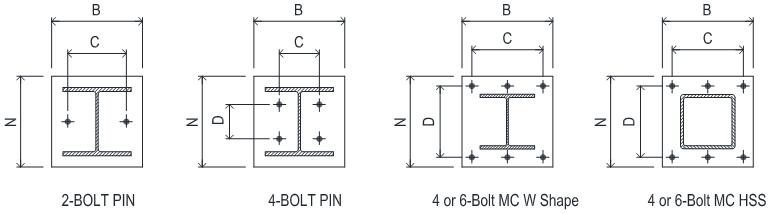Base plate width B = [mm] 510 mm
Base plate depth N = [mm] 510 mm
Base plate thickness tp = [mm] 45 mm
Anchor bolt spacing C C = [mm] 410 mm
Anchor bolt spacing D D = [mm] 410 mm
Concrete pedestal width bc = [mm]
Concrete pedestal depth dc = [mm]

Anchor bolt material   =
Anchor tensile strength futa = 399.9 [MPa]
Anchor bolt diameter da  = [mm] max dia=1.50 in
Anchor effective cross section area Ase = 625 [mm2]
Anchor bolt 1/8" (3mm) corrosion allowance =
Total no of anchor bolt in base plate n =
No of bolt along outermost bolt line nt =

Concrete strength fc' = [MPa]
Base plate yield strength Fy = [MPa]

 Load Cases Pu or Tu   [kN] Mu   [kN-m]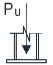LC1 Axial Compression Pu =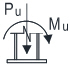LC2 Axial Compression + Moment Pu = Mu =LC3 Axial Compression + Moment Pu = Mu =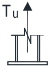LC4 Axial Tensile Tu =

Suggested plate thickness for rigidity:
tp = max. of m/4  and  n/4
=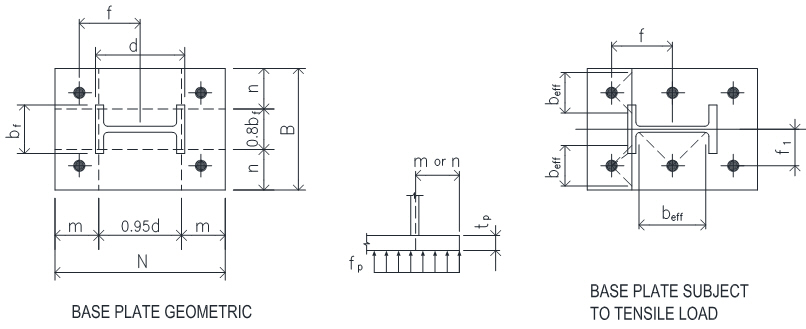Strength reduction factors                 CSA A23.3-14
Bearing on concrete fc = 0.65           8.4.2
Base plate bending fb = 0.90

CONCLUSION

Overall ratio = 0.75 OK
LC1: Axial Compression
Suggested Min Plate Thickness for Rigidity ratio = 0.75 OK
Pedestal Concrete Base Bearing Strength ratio = 0.06 OK
Base Plate Required Min Plan Size B x N ratio = 0.00 OK
Base Plate Required Min Thickness ratio = 0.37 OK
LC2: Axial Compression + Moment
Base Plate B x N or Anchor Bolt C x D Dimension Check ratio = 0.00 OK
Base Plate Required Min Thickness ratio = 0.69 OK
LC3: Axial Compression + Moment
Base Plate B x N or Anchor Bolt C x D Dimension Check ratio = 0.00 OK
Base Plate Required Min Thickness ratio = 0.43 OK
LC4: Axial Tension
Anchor Rod Tensile Resistance ratio = 0.16 OK
Base Plate B x N or Anchor Bolt C x D Dimension Check ratio = 0.00 OK
Base Plate Required Min Thickness - Bending to Flange/Web ratio = 0.35 OK

CACULATION                 AISC Design Guide 1
W Shape m = ( N - 0.95 d ) / 2 = 108.7 [mm] 3.1.2 on Page 15
n = ( B - 0.8 bf ) / 2 = 133.0 [mm]

Suggested min. plate thickness for rigidity: tp = max. of m/4 and n/4 = 33.3 [mm]
ratio = 0.75 < tp OK

LC1: Axial Compression
Concrete Base Bearing Strength
Factored compression force Pu =       = 444.8 [kN]
Base plate area A1 = B x N   = 2.6E+05 [mm2]
Pedestal area A2 = bc x dc   = 5.9E+05 [mm2]
CSA A23.3-14
k = min [ sqrt(A2/A1), 2 ] = 1.50   10.8.1
fc Pn = fc 0.85 fc' A1 k = 7717.0 [kN] 10.8.1
ratio = 0.06 > Pu OK

Base Plate Required Thickness             AISC Design Guide 1
X =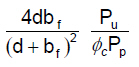= 0.058   3.1.2 on Page 16
l =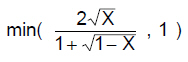= 0.244
l n' = l sqrt( d x bf ) / 4 = 18.7 [mm]
L = max (m, n, l n' ) = 133.0 [mm] 3.1.2 on Page 15
Base plate required thickness tmin =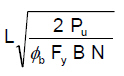= 16.5 [mm]
Base plate plan size B x N is adequate. ratio = 0.37 < tp OK

LC2: Axial Compression + Moment
Pu = 333.6 [kN] Mu = 67.8 [kN-m]
e = Mu / Pu = 203.2 [mm]
fp(max) = fc 0.85 fc' k = 29.7 [MPa]
qmax = fp(max) x B = 15131 [N/mm]
ecrit = N/2 - Pu / (2qmax) = 244.0 [mm]
e < ecrit small moment case applied

Small Moment Case
Bearing length Y = N - 2e = 103.5 [mm]
Verify linear bearing pressure q = Pu / Y = 3222 [N/mm]
< qmax OK
fp = Pu / BY = 6.3 [MPa]
m = max(m , n) = 133.0 [mm]
AISC Design Guide 1
Y < m tmin =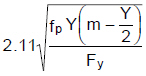= 30.9 [mm] Eq. 3.3.15a-1
Base plate plan size B x N is adequate. ratio = 0.69 < tp OK

LC3: Axial Compression + Moment
Pu = 200.2 [kN] Mu = 33.9 [kN-m]
e = Mu / Pu = 169.3 [mm]
fp(max) = fc 0.85 fc' k = 29.7 [MPa]
qmax = fp(max) x B = 15131 [N/mm]
ecrit = N/2 - Pu / (2qmax) = 248.4 [mm]
e < ecrit small moment case applied

Small Moment Case
Bearing length Y = N - 2e = 171.3 [mm]
Verify linear bearing pressure q = Pu / Y = 1168 [N/mm]
< qmax OK
fp = Pu / BY = 2.3 [MPa]
m = max(m , n) = 133.0 [mm]
AISC Design Guide 1
Y >= m tmin = 1.49 m sqrt( fp / Fy) = 19.0 [mm] Eq. 3.3.14a-1
Base plate plan size B x N is adequate. ratio = 0.43 < tp OK

LC4: Axial Tension
Factored tensile force Tu =       = 111.2 [kN]

Anchor Rod Tensile Resistance                 CSA A23.3-14
Anchor rod tensile resistance Tr = fs n Ase futa Rt,s = 680.0 [kN] D.6.1.2  Eq D.2
fs = 0.85   Rt,s = 0.80   8.4.3 a),  D.5.3 a)
ratio = 0.16 > Tu OK

Bolt pattern Bolt Both Outside and Inside Flange
Total No of anchor bolt n =       = 4.0
Bolt to column center distance f =       = 205 [mm]
Bolt to column web center distance f1 =       = 205 [mm]
Factored tensile force in single bolt Tb = Tu / n = 27.8 [kN]Bending to Column Flange                 AISC Design Guide 1
Moment lever arm a = f - 0.5 d + 0.5 tf = 59 [mm] Eq 3.4.6  on Page 26
Moment to column flange Mu = Tb x a = 1.6 [kN-m]
Effective plate width beff = 2 x a = 117 [mm] Sect 3.2 3 on Page 18

Base plate required thickness tp1 =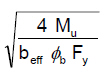= 15.8 [mm] Eq 3.3.13a  on Page 25

Bending to Column Web                 AISC Design Guide 1
Moment lever arm a = f1 - 0.5 tw = 200 [mm] Example 4.5 on Page 34
Moment to column web Mu = Tb x a = 5.6 [kN-m]
Effective plate width beff = 2 x a = 400 [mm] Example 4.5 on Page 35

Base plate required thickness tp2 == 15.8 [mm] Example 4.5 on Page 35
tmin = max ( tp1, tp2 ) = 15.8 [mm]
Base plate plan size B x N is adequate. ratio = 0.35 < tp OK

 ToolTip OnOff